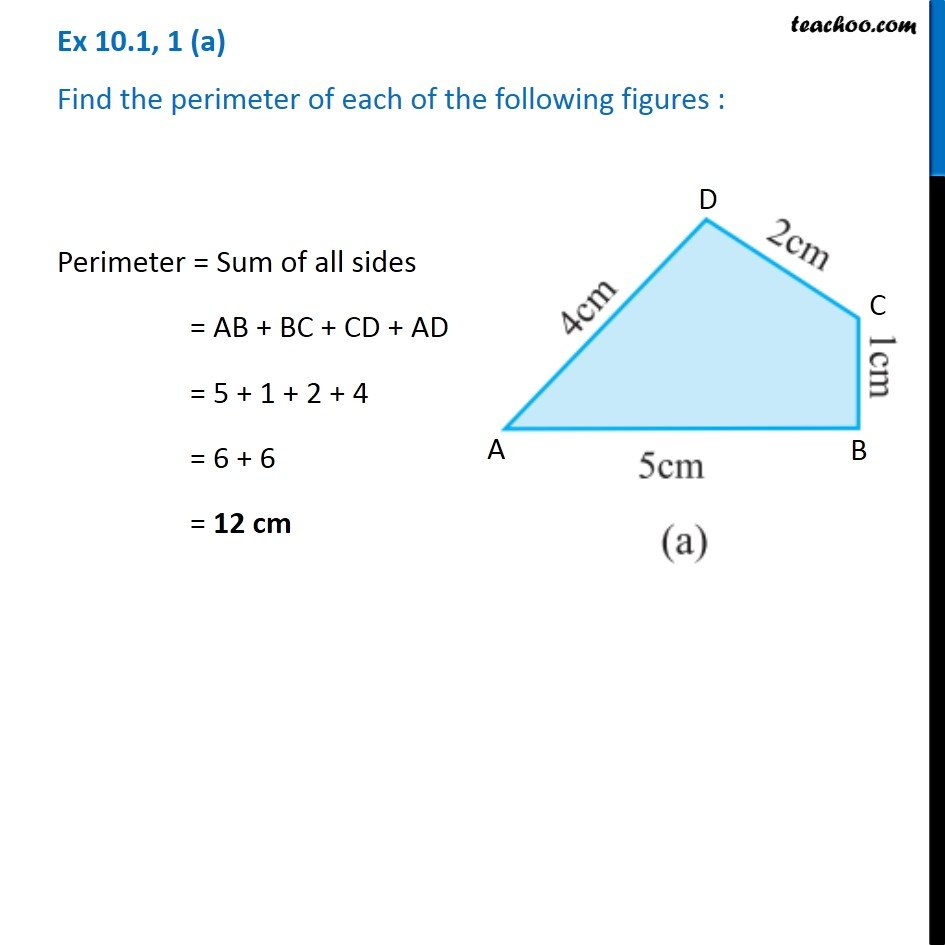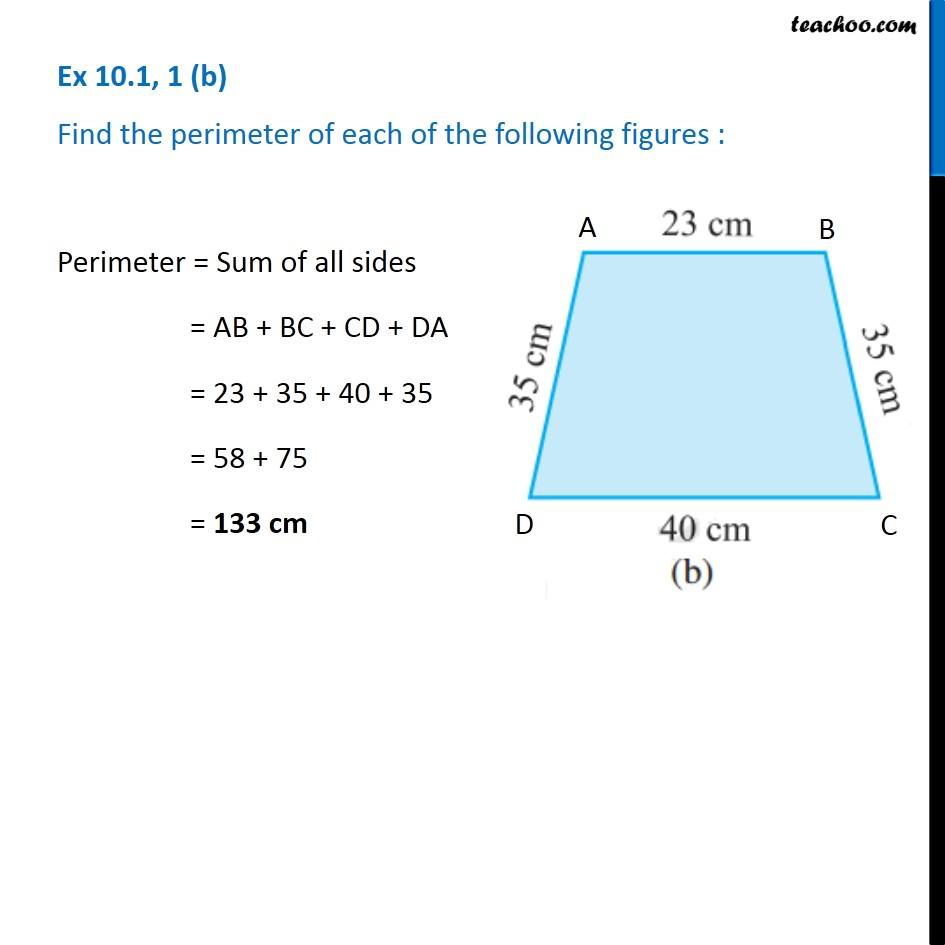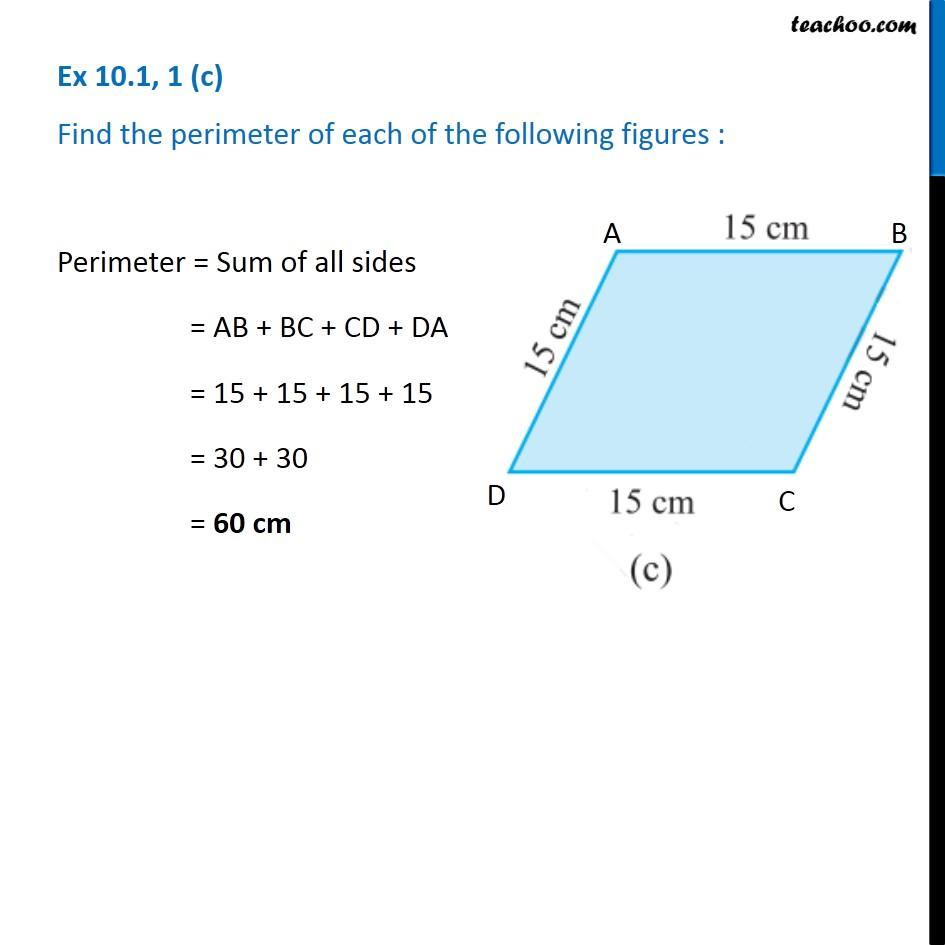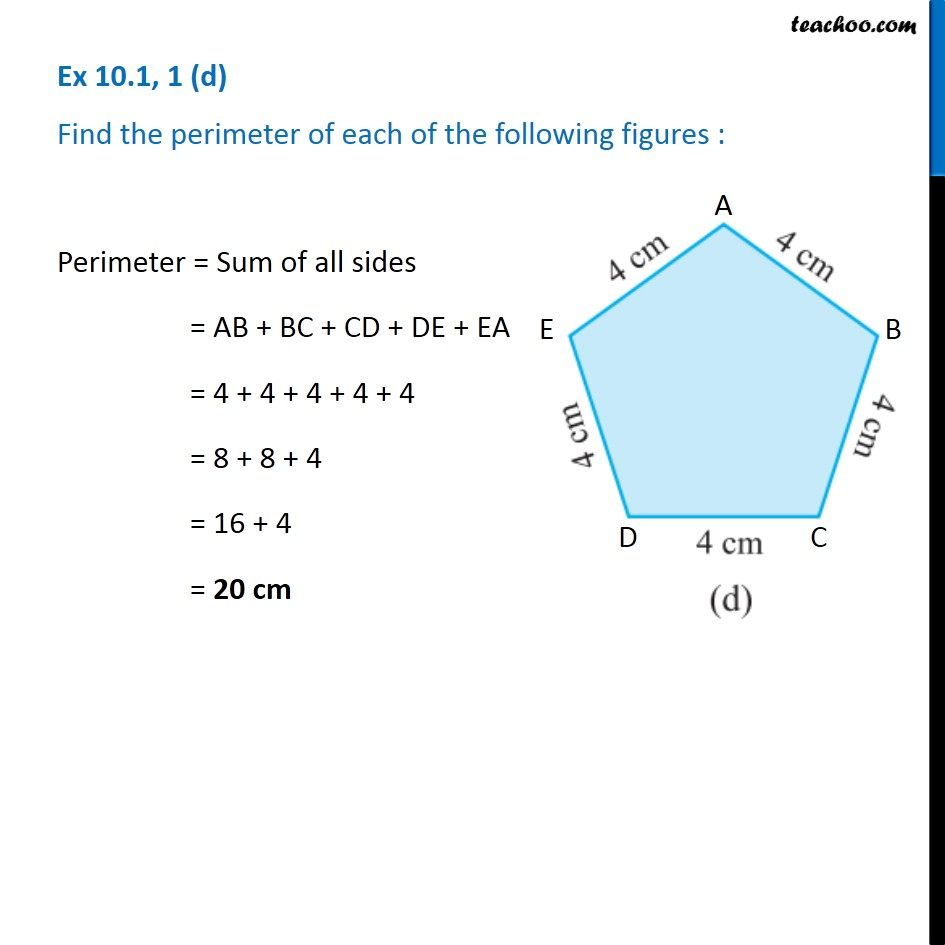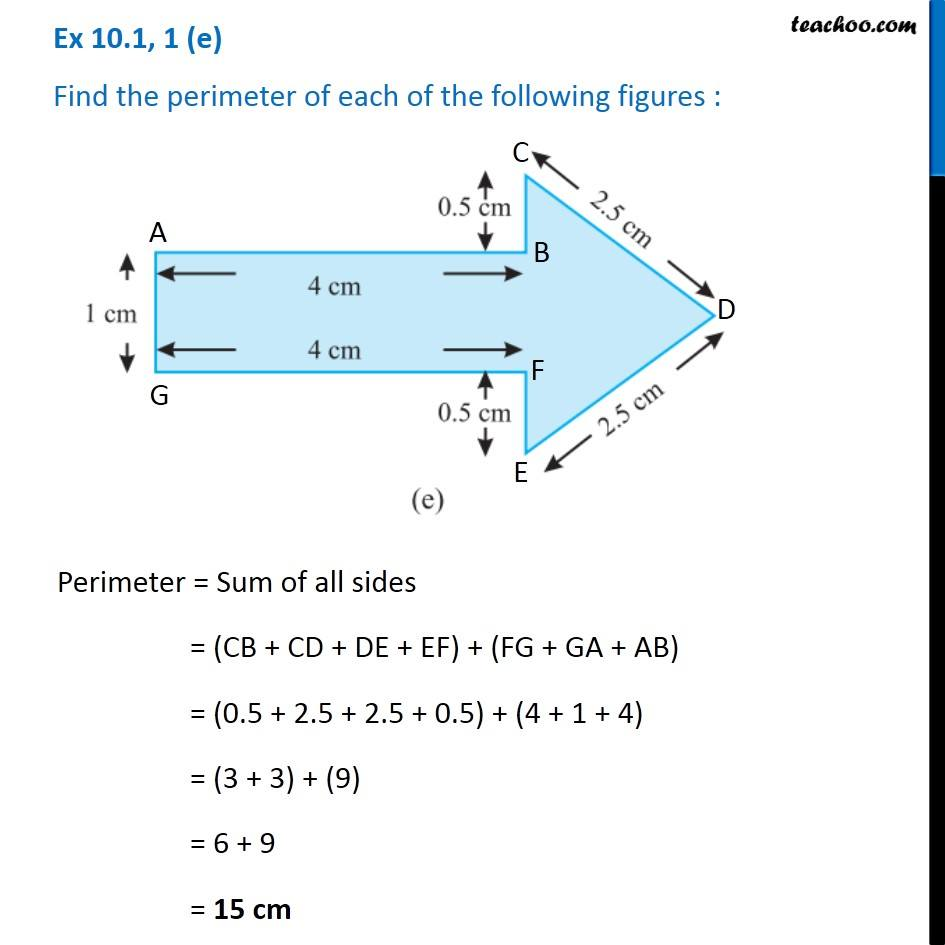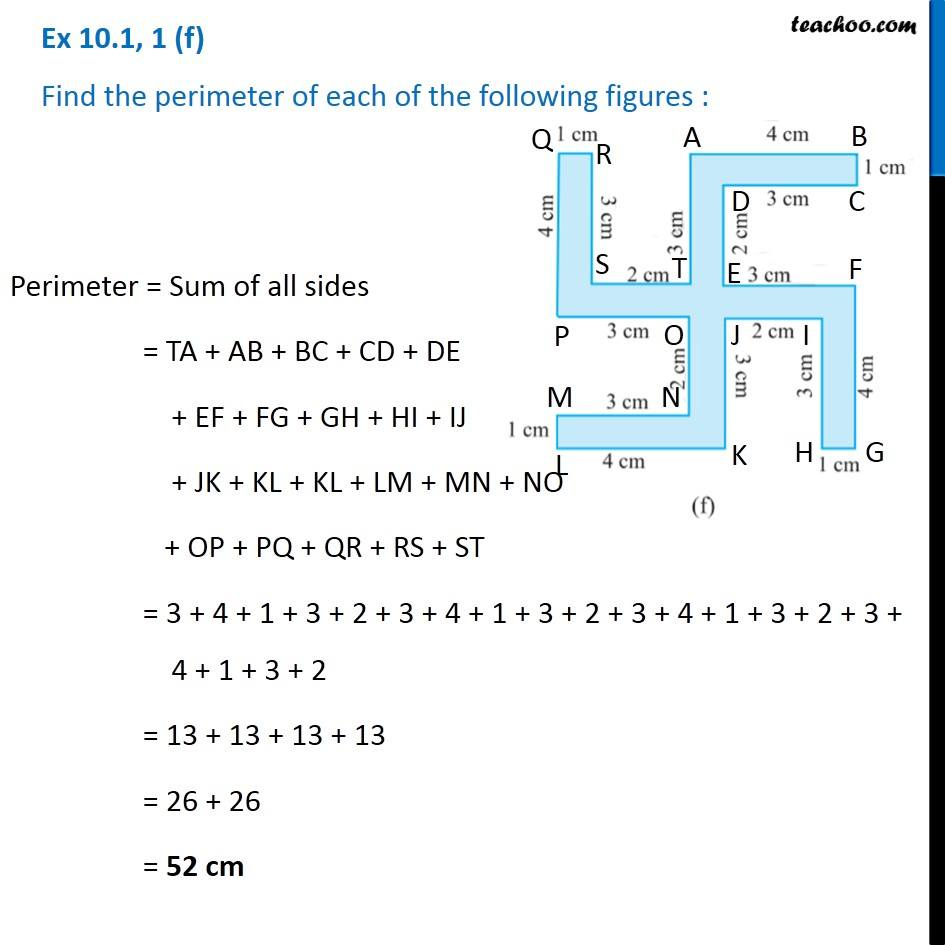1. Chapter 10 Class 6 Mensuration
2. Serial order wise
3. Ex 10.1

Transcript

Ex 10.1, 1 (a) Find the perimeter of each of the following figures : Perimeter = Sum of all sides = AB + BC + CD + AD = 5 + 1 + 2 + 4 = 6 + 6 = 12 cm Ex 10.1, 1 (b) Find the perimeter of each of the following figures : Perimeter = Sum of all sides = AB + BC + CD + DA = 23 + 35 + 40 + 35 = 58 + 75 = 133 cm Ex 10.1, 1 (c) Find the perimeter of each of the following figures : Perimeter = Sum of all sides = AB + BC + CD + DA = 15 + 15 + 15 + 15 = 30 + 30 = 60 cm Ex 10.1, 1 (d) Find the perimeter of each of the following figures : Perimeter = Sum of all sides = AB + BC + CD + DE + EA = 4 + 4 + 4 + 4 + 4 = 8 + 8 + 4 = 16 + 4 = 20 cm Ex 10.1, 1 (e) Find the perimeter of each of the following figures : Perimeter = Sum of all sides = (CB + CD + DE + EF) + (FG + GA + AB) = (0.5 + 2.5 + 2.5 + 0.5) + (4 + 1 + 4) = (3 + 3) + (9) = 6 + 9 = 15 cm Ex 10.1, 1 (f) Find the perimeter of each of the following figures : Perimeter = Sum of all sides = TA + AB + BC + CD + DE + EF + FG + GH + HI + IJ + JK + KL + KL + LM + MN + NO + OP + PQ + QR + RS + ST = 3 + 4 + 1 + 3 + 2 + 3 + 4 + 1 + 3 + 2 + 3 + 4 + 1 + 3 + 2 + 3 + 4 + 1 + 3 + 2 = 13 + 13 + 13 + 13 = 26 + 26 = 52 cm

Ex 10.1